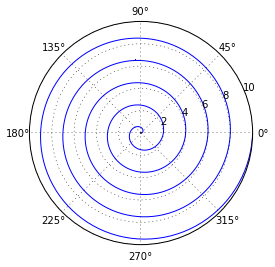# 3. Various Plotting Examples¶

Use the “v2” files in older versions of IPython, e.g. 0.12

# Matplotlib Tutorial: 3. Useful Plot Types

So far we have dealt with simple line and point plots using the plot command. There are a wide array of other plot types available in matplotlib; we'll explore a few of them here. An excellent reference for this is the Plotting Commands Summary in the matplotlib documentation. For a more visual summary of available routines, see the Gallery Page.

As always, we start with entering pylab mode and doing some imports

In :
%pylab inline

# The following imports are not strictly necessary: they
# are done by default with the above pylab mode command
import numpy as np
import matplotlib.pyplot as plt

Welcome to pylab, a matplotlib-based Python environment [backend: module://IPython.zmq.pylab.backend_inline].


## Histograms

Histograms can be used to judge the density of 1-dimensional data. For example:

In :
x = np.random.normal(size=1000)

fig, ax = plt.subplots()

H = ax.hist(x, bins=50, alpha=0.5, histtype='stepfilled')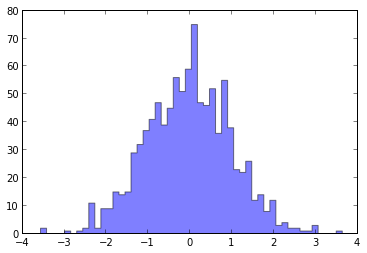## Pie Plot

Matplotlib can create pie diagrams with the function pie:

In :
fracs = [30, 15, 45, 10]
colors = ['b', 'g', 'r', 'w']

fig, ax = plt.subplots(figsize=(6, 6))  # make the plot square
pie = ax.pie(fracs, colors=colors, explode=(0, 0, 0.05, 0), shadow=True,
labels=['A', 'B', 'C', 'D'])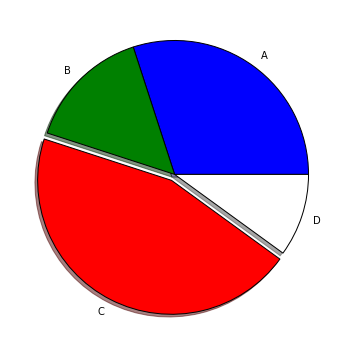## Errorbar Plots

Often we want to add errorbars to our points. The errorbar function works in a similar way to plot, but adds vertical and/or horizontal errorbars to the points.

In :
x = np.linspace(0, 10, 30)
dy = 0.1
y = np.random.normal(np.sin(x), dy)

fig, ax = plt.subplots()
plt.errorbar(x, y, dy, fmt='.k')

Out :
<Container object of 3 artists>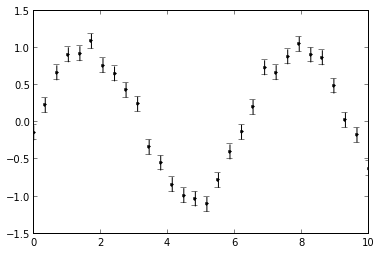## Filled Plots

Sometimes you'd like to fill the region below a curve, or between two curves. The functions fill and fill_between can be very useful for this:

In :
x = np.linspace(0, 10, 1000)
y1 = np.sin(x)
y2 = np.cos(x)

fig, ax = plt.subplots()
ax.fill_between(x, y1, y2, where=(y1 < y2), color='red')
ax.fill_between(x, y1, y2, where=(y1 > y2), color='blue')

Out :
<matplotlib.collections.PolyCollection at 0x2b60d90>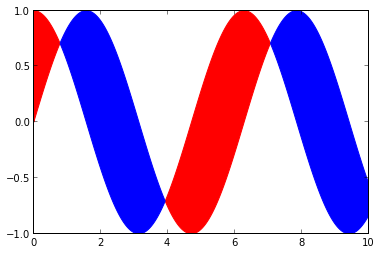## Scatter Plots

We have seen scatterplots before, when using point-type line styles in the plot command. The scatter command allows more flexibility in the colors and shapes of the points:

In :
x = np.random.random(50)
y = np.random.random(50)
c = np.random.random(50)  # color of points
s = 500 * np.random.random(50)  # size of points

fig, ax = plt.subplots()
im = ax.scatter(x, y, c=c, s=s, cmap=plt.cm.jet)

# Add a colorbar
fig.colorbar(im, ax=ax)

# set the color limits - not necessary here, but good to know how.
im.set_clim(0.0, 1.0)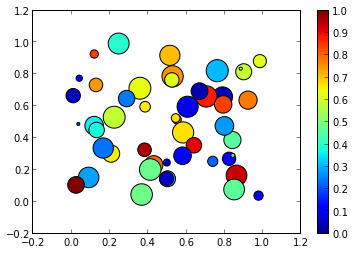## Contour Plots

Contour plots can be used to show the variation of a quantity with respect to two others:

In :
x = np.linspace(0, 10, 50)
y = np.linspace(0, 20, 60)

z = np.cos(y[:, np.newaxis]) * np.sin(x)

fig, ax = plt.subplots()

# filled contours
im = ax.contourf(x, y, z, 100)

# contour lines
im2 = ax.contour(x, y, z, colors='k')

fig.colorbar(im, ax=ax)

Out :
<matplotlib.colorbar.Colorbar instance at 0x352f5a8>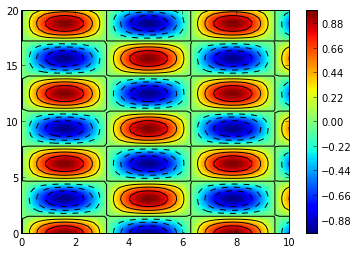## Showing Images

The imshow command allows displaying images in a variety of formats. It can be useful for actual image data, as well as being useful for visualizing datasets in a way similar to the contour plots above.

In :
I = np.random.random((100, 100))

I += np.sin(np.linspace(0, np.pi, 100))

fig, ax = plt.subplots()

im = ax.imshow(I, cmap=plt.cm.jet)

fig.colorbar(im, ax=ax)

Out :
<matplotlib.colorbar.Colorbar instance at 0x2da8710>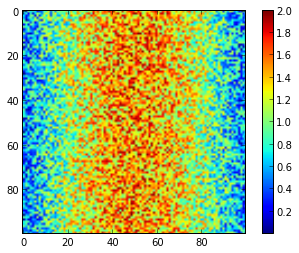## 2D Histograms and Hexbin

hist2D and hexbin are ways to represent binned two-dimensional data. They can be used as follows:

In :
x, y = np.random.normal(size=(2, 10000))

fig, ax = plt.subplots()
im = ax.hexbin(x, y, gridsize=20)
fig.colorbar(im, ax=ax)

fig, ax = plt.subplots()
H = ax.hist2d(x, y, bins=20)
fig.colorbar(H, ax=ax)

Out :
<matplotlib.colorbar.Colorbar instance at 0x3b57e60>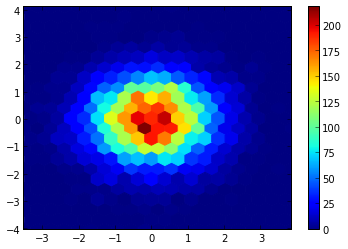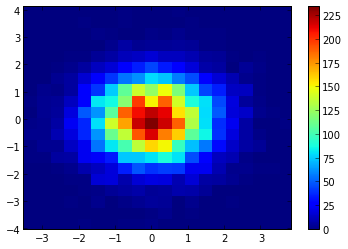## Polar Plots

It is also possible to plot data in coordinates other than Cartesian. Here we'll show how to do a polar plot

In :
fig = plt.figure()
ax = fig.add_subplot(1, 1, 1, projection='polar')

theta = np.linspace(0, 10 * np.pi, 1000)
r = np.linspace(0, 10, 1000)

ax.plot(theta, r)

Out :
[<matplotlib.lines.Line2D at 0x2626fd0>]